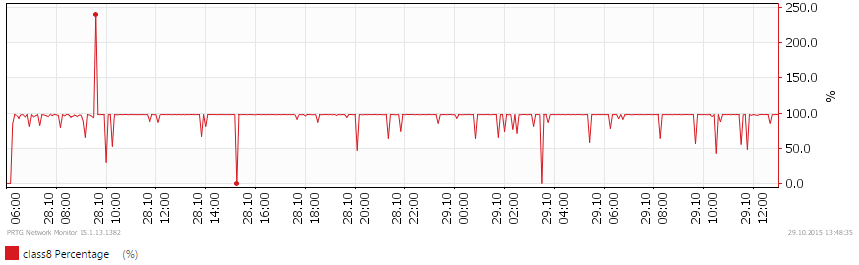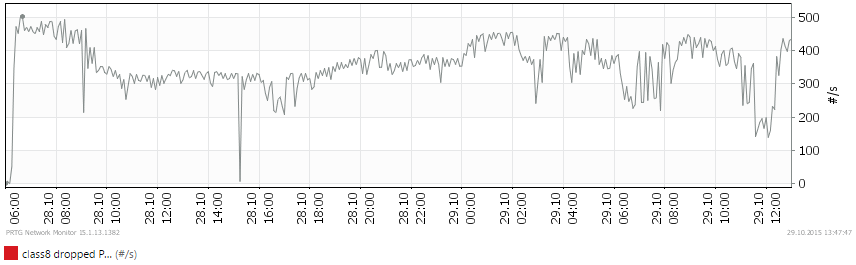Highlighted

## QoS Monitoring (for PRTG Network Monitor)

Hi all,

Because there are no useful methods to monitor the configured QoS values (at least til 7.0.3, I don't know if there will be a monitoring of QoS in the future), I have written a little script in powershell which gets all the required values over the XML API and the creates an output which could be used for the software PRTG Network Monitor (there is also a free version with limited amount of sensors).

The syntax looks like the following:

"script.ps1 <fwhostname> <physicalinterface> [<qosrule/tunnelinterface>] [tunnel-traffic]"

example for a cleartext rule named "Traffic-to-Internet" on ethernet1/3:

script.ps1 "firewall.domain.local" "ethernet1/3" "Traffic-to-Internet"

example for monitoring a tunnel-traffic rule for tunnel 10 on ethernet1/2:

script.ps1 "firewall.domain.local" "ethernet1/2" "tunnel.10" "tunnel-traffic"

If someone is interested, you can find the script here: https://github.com/inaxis/Palo_QoS_Mon/blob/master/Palo_QoS_Mon_Script.ps1

But you have to be carefully and do not run too many instances of the script at the same time towards a single firewall (specially not a pa-2000), or if you do it have a look at the management cpu utilization.

I tested the script on the following device/software combinations:

• PA-5050 (PAN-OS 6.1.7)
• PA-5020 (PAN-OS 7.0.3)
• PA-3020 (PAN-OS 6.1.7)

The output of the devices is slightly different, so the channel which shows the dropped packets/second does not work on PA-5000 series.

Regards,

Remo

Example screenshots:

Actual bandwidth:Utilization in percent:Dropped packets/second:Tags (5)

## Re: QoS Monitoring (for PRTG Network Monitor)

Hello, I think your post is interesting, but I also have a question about: in what language is it written and how to put it together with PRTG Network Monitor?

## Re: QoS Monitoring (for PRTG Network Monitor)

The script is written in powershell. To make it work you need to place the script in the PRTG folder for custom scripts and then you need to create a new "EXE/Script Advanced" sensor and add the parameters as needed into the corresponding inputfield on the create sensor page.

Would be cool to know someone will actually use my solution ;)

## Re: QoS Monitoring (for PRTG Network Monitor)

- Where I need to apply syntax ?

- What paramater should I put to moinitor qos all class ?

## Re: QoS Monitoring (for PRTG Network Monitor)

@chaopov

What PAN-OS Version do you use? (Because of some changes in the API my script needs some adjustments for PAN-OS 8)

## Re: QoS Monitoring (for PRTG Network Monitor)

- I use PAN-OS 8.0.10.

- I have modify script as below

- In PRTG I have add XML EXE/Script Sensor. and PRTG show qos class channel already but didn't show realtime bandwidth per qos class.

```param(
[string]\$fw ,
[string]\$if ,
[string]\$rule = "regular-traffic",
[string]\$type = "regular-traffic")

[System.Net.ServicePointManager]::ServerCertificateValidationCallback = {\$true}
[Net.ServicePointManager]::SecurityProtocol =
[Net.SecurityProtocolType]::Tls12

Function callApiOp (\$fw, \$param)
{
# Function to call an operational-api action
\$request = New-Object System.Net.WebClient
\$apiurl = "https://" + \$fw + "/api/?key=" + \$api_login_string + "&type=op&cmd=" + "<show><qos><interface></interface></qos></show>"

}

Function getConfig (\$fw, \$xp)
{
# Function to call a config-api action
\$request = New-Object System.Net.WebClient
\$apiurl = "https://" + \$fw + "/api/?key=" + \$api_login_string + "&type=config&action=get&xpath=" + \$xp
return [xml]\$xml.response.result.innerXML
}

#Get the QoS configuration of the firewall
\$xp = getConfig \$fw "/config/devices/entry[@name='localhost.localdomain']/network/qos"
#xp = callApiOp \$fw "<show><config><running></running></config></show>"
#\$param = callApiOp \$fw "<show><qos><interface><entry name=\$if><throughtput>0</throughtput></entry></interface></qos></show>"

#Extracte the QoS configuration for the supplied interface
\$qosinterface = \$xp.qos.interface.entry | Where {\$_.name -eq \$if}

#Check if a rule was supplied, if yes extract the configuration of that particular rule
if (\$rule -ne "regular-traffic")
{
\$qosRegularRule = \$qosinterface.\$type.groups.entry.members.entry | Where {\$_.name -eq \$rule}
}
#If the variable \$rule is equal to \$null then set the following variables
else
{
\$qosRegularRule = "regular"
\$rule = "regular-traffic"
\$rulenumber = 0
}

#Set the xml header and expected prtg root tag
\$prtgoutput = '<?xml version="1.0" encoding="Windows-1252" ?>'
\$prtgoutput += "<prtg>`n"

#Check if either the interfaceconfig or the ruleconfig is empty
if (\$qosinterface -eq \$null)
{
\$prtgoutput += "<error>1</error>`n"
\$prtgoutput += "<text>Invalid interface</text>`n"
\$prtgoutput += "</prtg>`n"
\$prtgoutput
exit
}
if (\$qosRegularRule -eq \$null)
{
\$prtgoutput += "<error>1</error>`n"
\$prtgoutput += "<text>Invalid QoS Rule</text>`n"
\$prtgoutput += "</prtg>`n"
\$prtgoutput
exit
}

#Additionally, check if QoS is enabled on the supplied interface
if (\$xp.SelectSingleNode("/qos/interface/entry[@name='\$if']/enabled").get_InnerXML() -ne "yes")
{
\$prtgoutput += "<error>1</error>`n"
\$prtgoutput += "<text>QoS disabled on this interface</text>`n"
\$prtgoutput += "</prtg>"
\$prtgoutput
exit
}

if (\$rulenumber -ne 0)
{
#Create an empty hashtable for storing a mappingtable for Qos rulenumber (=node ID) and the rulename
\$rulearray = @{}

#Count through the regular rules and add their numbers and names to the hashtable
\$count = 1
foreach (\$regularrule in \$qosinterface.'regular-traffic'.groups.entry.members.entry)
{
\$count++
}
#Get the Node ID for the supplied rulename
\$rulenumber = \$rulearray[\$rule].tostring()
}

#Get the output of the command "show qos interface <ifname> throughput <nodeid> or for the supplied tunnelinterface"
switch (\$type)
{
"regular-traffic"
{
\$traffic = callApiOp \$fw "<show><qos><interface><entry name=\$if><throughput>\$rulenumber</throughput></entry></interface></qos></show>"
}
"tunnel-traffic"
{
\$traffic = callApiOp \$fw "<show><qos><interface><entry name=\$if><tunnel-throughput>\$rule</tunnel-throughput></entry></interface></qos></show>"
}
default
{
\$prtgoutput += "<error>1</error>`n"
\$prtgoutput += "<text>Wrong traffic type. Leave blank for 'regular-traffic' or enter 'tunnel-traffic'</text>`n"
\$prtgoutput += "</prtg>"
\$prtgoutput
exit
}
}

#Reduce the output to an array of max. 8 values which should only contain bandwidth values in kbps
\$regex = '([cC]lass\s\d[:\s]\s{0,}\d{0,}\skbps)'
\$traffic = \$traffic.response.result | Select-String -Pattern \$regex -AllMatches | % { \$_.Matches } | % { \$_.Value }

#Replace special characters, which need to be escaped in a regex string
\$temprule = \$rule.Replace(".","\.").Replace(" ","\s")
#Regex string which extracts the needed information from the command "show qos interface <IF> counter"
\$counterRegex = '(\s{1,}\d{1,}\s{1,}\d{1,}\s' + \$temprule + '\s{1,}\d{1,}\s{1,}\d{1,}\s{1,}\d{1,}\s{1,}\d{1,}\s{1,}\d{1,}\s{1,}\d{1,}\s{1,}\d{1,}\s{1,}\d{1,}\s{1,}\d{1,}\n((?:\s{1,}-Class\s{1,}\d{1}\s{1,}\d{1,}\s{1,}\d{1,}\s{1,}\d{1,}\s{1,}\d{1,}\s{1,}\d{1,}\s{1,}\d{1,}\s{1,}\d{1,}\s{1,}\d{1,}\s{1,}\d{1,}\s{1,}\d{1,3}\n)+)|\s{1,}\d{1,}\s{1,}\d{1,}\s' + \$temprule + '\s{1,}\d{1,}\s{1,}\d{1,}\s{1,}\d{1,}\s{1,}\d{1,}\s{1,}\d{1,}\s{1,}\d{1,}\s{1,}\d{1,}\s{1,}\d{1,}\s{1,}\d{1,}\n)'
\$qoscounter = callApiOp \$fw "<show><qos><interface><entry name=\$if><counter></counter></entry></interface></qos></show>"
\$qoscounter = \$qoscounter.response.result  | Select-String -Pattern \$counterRegex | % { \$_.Matches } | % { \$_.Value }

#Create sensors for every QoS class
for (\$i = 1; \$i -le 8; \$i++)
{
\$regex = '([cC]lass\s' + \$i.tostring() + '[:\s]\s{0,}\d{0,}\skbps)'
\$line = \$traffic | Select-String -Pattern \$regex | % { \$_.Matches } | % { \$_.Value }
\$maxbandwidth = \$null
\$egressguaranteed = \$null

if (\$line -eq \$null)
{
\$trafficvalue = 0
\$class = \$i
\$classname = "class" + \$class.ToString()
}
else
{
#convert the \$line variable with the bandwith to an integer variable
\$trafficvalue = \$line | Select-String -Pattern '(\d*\skbps)' | % { \$_.Matches } | % { \$_.Value } | Select-String -Pattern '(\d*)' | % { \$_.Matches } | % { \$_.Value }
\$trafficvalue = [int]\$trafficvalue
\$class = \$line | Select-String -Pattern '([cC]lass\s\d)' | % { \$_.Matches } | % { \$_.Value } | Select-String -Pattern '(\d)' | % { \$_.Matches } | % { \$_.Value }
\$classname = "class" + \$class.ToString()
}
[decimal]\$bps = \$trafficvalue * 1024
[decimal]\$kbps = \$trafficvalue
[decimal]\$mbps = \$trafficvalue / 1024

#Get the applied QoS Profilename if a rulename was supplied or get the profile for default profile for regular/tunnel traffic
if (\$rule -ne "regular-traffic")
{
\$qosProfileName = \$xp.SelectSingleNode("/qos/interface/entry [@name='\$if']/\$type/groups/entry [@name='\$type-group']/members/entry [@name='\$rule']/qos-profile").get_InnerXML()
}
else
{
\$qosProfileName = \$qosinterface.\$type.'default-group'.InnerText
}

#Check if a maximum egress value was set in the profile for the specific class
if (\$xp.SelectSingleNode("/qos/profile/entry [@name='\$qosProfileName']/class/entry [@name='\$classname']/class-bandwidth/egress-max") -ne \$null)
{
try {
[int32]\$maxbandwidth = \$xp.SelectSingleNode("/qos/profile/entry [@name='\$qosProfileName']/class/entry [@name='\$classname']/class-bandwidth/egress-max").get_InnerXML()
}
catch {
\$maxbandwidth = \$null
}
}

#Check if the max-egress value was empty or zero; if yes get the aggregate max-egress value from the QoS Profile
if ((\$maxbandwidth -eq \$null) -or (\$maxbandwidth -eq 0))
{
try {
[int32]\$maxbandwidth = \$xp.SelectSingleNode("/qos/profile/entry [@name='\$qosProfileName']/aggregate-bandwidth/egress-max").get_InnerXML()
}
catch {
\$maxbandwidth = \$null
}

#Check if the aggregate max-egress value was empty or zero; if yes get the max-egress value for regular traffic on that interface
if ((\$maxbandwidth -eq \$null) -or (\$maxbandwidth -eq 0))
{
try {
[int32]\$maxbandwidth = \$qosinterface.\$type.bandwidth.'egress-max'
}
catch {
\$maxbandwidth = \$null
}

#Check if the regular traffic max-egress value was empty or zero; if yes get the max-egress value of the interface
if ((\$maxbandwidth -eq \$null) -or (\$maxbandwidth -eq 0))
{
try {
[int32]\$maxbandwidth = \$qosinterface.'interface-bandwidth'.'egress-max'
}
catch {
\$maxbandwidth = \$null
}

#Check if the max-egress value on the interface is empty or zero; if yes get the speed value of the physical interface
if ((\$maxbandwidth -eq \$null) -or (\$maxbandwidth -eq 0))
{
\$op = "<show><interface>" + \$if + "</interface></show>"
\$interface = callApiOp \$fw \$op
[int32]\$maxbandwidth = \$interface.response.result.hw.speed
}
}
}
}

#Check if a guaranteed egress value was set in the profile for the specific class
if (\$xp.SelectSingleNode("/qos/profile/entry [@name='\$qosProfileName']/class/entry [@name='\$classname']/class-bandwidth/egress-guaranteed") -ne \$null)
{
try {
[int32]\$egressguaranteed = \$xp.SelectSingleNode("/qos/profile/entry [@name='\$qosProfileName']/class/entry [@name='\$classname']/class-bandwidth/egress-guaranteed").get_InnerXML()
}
catch {
\$egressguaranteed = \$null
}
}

#Check if the guaranteed-egress value was empty or zero; if yes get the aggregate guaranteed-egress value from the QoS Profile
if ((\$egressguaranteed -eq \$null) -or (\$egressguaranteed -eq 0))
{
try {
[int32]\$egressguaranteed = \$xp.SelectSingleNode("/qos/profile/entry [@name='\$qosProfileName']/aggregate-bandwidth/egress-guaranteed").get_InnerXML()
}
catch {
\$egressguaranteed = \$null
}

#Check if the aggregate guaranteed-egress value was empty or zero; if yes get the guaranteed-egress value for regular traffic on that interface
if ((\$egressguaranteed -eq \$null) -or (\$egressguaranteed -eq 0))
{
try {
[int32]\$egressguaranteed = \$qosinterface.\$type.bandwidth.'egress-guaranteed'
}
catch {
\$egressguaranteed = \$null
}
}
}

\$prtgoutput += "<result>`n"
\$prtgoutput += "<channel>" + \$classname +"</channel>`n"
\$prtgoutput += "<unit>Custom</unit>`n"
\$prtgoutput += "<customunit>Mbit/s</customunit>`n"
\$prtgoutput += "<mode>Absolute</mode>`n"
\$prtgoutput += "<showChart>1</showChart>`n"
\$prtgoutput += "<showTable>1</showTable>`n"
\$prtgoutput += "<float>1</float>`n"
\$prtgoutput += "<value>" + \$mbps.ToString() + "</value>`n"
\$prtgoutput += "<LimitMode>1</LimitMode>`n"
\$prtgoutput += "<LimitMaxError>" + \$maxbandwidth.ToString() + "</LimitMaxError>`n"
\$prtgoutput += "<LimitErrorMsg>The bandwidth  of " + \$classname + " exeeds the configured max. value of " + \$maxbandwidth.ToString() + " mbps</LimitErrorMsg>`n"

#if there is a value for egress-guaranteed and it is not 0 then create a warning-limit in the traffic-channel and an additional sensor for making this limit visible in the chart
if (\$egressguaranteed -ne \$null -and \$egressguaranteed -ne 0)
{
\$prtgoutput += "<LimitMaxWarning>" + \$egressguaranteed.ToString() + "</LimitMaxWarning>`n"
\$prtgoutput += "<LimitWarningMsg>The bandwidth of " + \$classname + " exeeds the configured guaranteed value of " + \$egressguaranteed.ToString() + " mbps</LimitWarningMsg>`n"
\$prtgoutput += "</result>`n"

\$prtgoutput += "<result>`n"
\$prtgoutput += "<channel>" + \$classname +" egress-guaranteed</channel>`n"
\$prtgoutput += "<unit>Custom</unit>`n"
\$prtgoutput += "<customunit>Mbit/s</customunit>`n"
\$prtgoutput += "<mode>Absolute</mode>`n"
\$prtgoutput += "<showChart>1</showChart>`n"
\$prtgoutput += "<showTable>0</showTable>`n"
\$prtgoutput += "<float>1</float>`n"
\$prtgoutput += "<value>" + \$egressguaranteed.ToString() + "</value>`n"
\$prtgoutput += "</result>`n"
}
else
{
\$prtgoutput += "</result>`n"
}

\$prtgoutput += "<result>`n"
\$prtgoutput += "<channel>" + \$classname +" egress-max</channel>`n"
\$prtgoutput += "<unit>Custom</unit>`n"
\$prtgoutput += "<customunit>Mbit/s</customunit>`n"
\$prtgoutput += "<mode>Absolute</mode>`n"
\$prtgoutput += "<showChart>1</showChart>`n"
\$prtgoutput += "<showTable>0</showTable>`n"
\$prtgoutput += "<float>1</float>`n"
\$prtgoutput += "<value>" + \$maxbandwidth.ToString() + "</value>`n"
\$prtgoutput += "</result>`n"

#Calculate the used bandwidth in percent
\$percent = (\$mbps / \$maxbandwidth) * 100

\$prtgoutput += "<result>`n"
\$prtgoutput += "<channel>" + \$classname +" Percentage</channel>`n"
\$prtgoutput += "<unit>Percent</unit>`n"
\$prtgoutput += "<mode>Absolute</mode>`n"
\$prtgoutput += "<showChart>1</showChart>`n"
\$prtgoutput += "<showTable>1</showTable>`n"
\$prtgoutput += "<float>1</float>`n"
\$prtgoutput += "<value>" + \$percent + "</value>`n"
\$prtgoutput += "</result>`n"

\$regex = 'Class\s' + \$class.toString() + '\s{1,}\d{1,}\s{1,}\d{1,}\s{1,}\d{1,}\s{1,}\d{1,}\s{1,}(\d{1,12})\s{1,}\d{1,}\s{1,}\d{1,}\s{1,}\d{1,}\s{1,}\d{1,}\s{1,}\d{1,3}'
\$droppedPackets = \$qoscounter | Select-String -Pattern \$regex | % { \$_.Matches } | % { \$_.Value }
if (\$droppedPackets -ne \$null)
{
\$regex = 'Class\s' + \$class.toString() + '\s{1,}\d{1,}\s{1,}\d{1,}\s{1,}\d{1,}\s{1,}\d{1,}\s{1,}'
\$droppedPackets = \$droppedPackets -replace \$regex,'' | Select-String -Pattern '\d{1,}' | % { \$_.Matches } | % { \$_.Value }
\$prtgoutput += "<result>`n"
\$prtgoutput += "<channel>" + \$classname +" dropped Packets</channel>`n"
\$prtgoutput += "<unit>#</unit>`n"
\$prtgoutput += "<mode>Difference</mode>`n"
\$prtgoutput += "<showChart>1</showChart>`n"
\$prtgoutput += "<showTable>1</showTable>`n"
\$prtgoutput += "<float>0</float>`n"
\$prtgoutput += "<value>" + \$droppedPackets + "</value>`n"
\$prtgoutput += "</result>`n"
}
}

#Close the xml root tag and show the output
\$prtgoutput += "</prtg>"
\$prtgoutput```## Re: QoS Monitoring (for PRTG Network Monitor)

trying to implement this and am able to get it working in a testing environment, but in prod we have the PA's managed by Panorama.  For some reason when calling the config using the api, I dont get the ethernet names, ie ethernet1/1, so it breaks the PS script.  Is someone able to point out as to why the ethernets are not showing?

looking into the PS script, it is written as <show><qos><interface></interface></qos></show>, i get a status="error"

when i manually call same thing, but if i add the addition xml of  <show><qos><interface><entry name="ethernet1/1"><show-bypass-node/></entry></interface></qos></show>, i can see the data no problem

Like what you see?